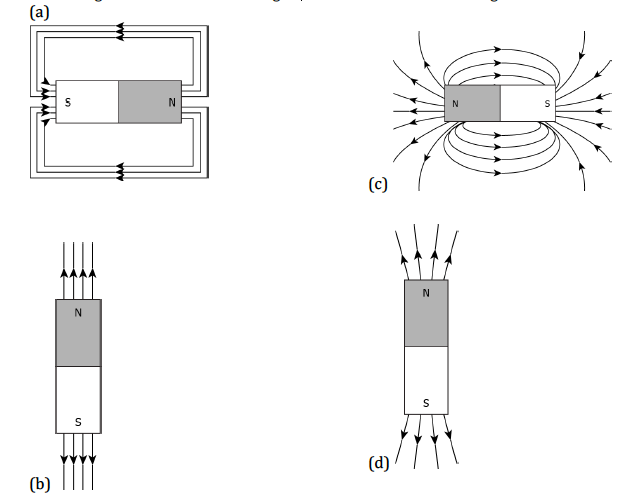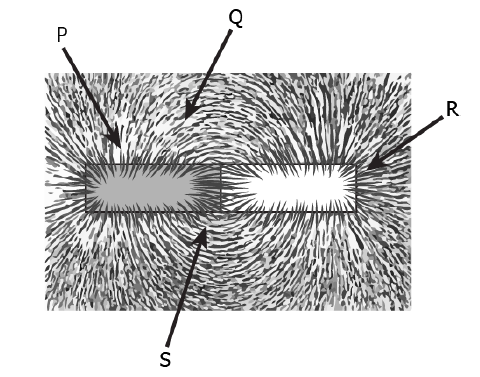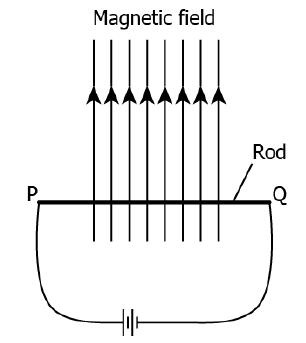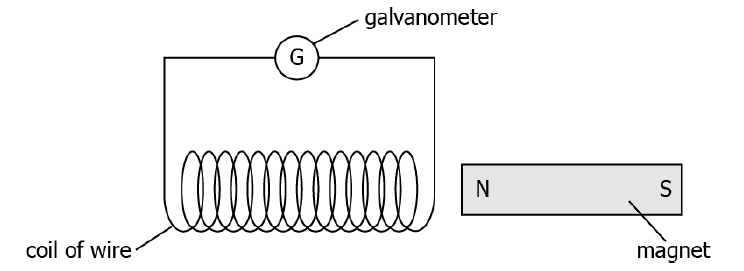# CBSE Class 10 Science MCQ Chapter 13 Magnetic Effects of Electric Current

## Magnetic Effects of Electric Current Class 10 MCQ

CBSE Class 10 Science MCQ Chapter 13 Magnetic Effects of Electric Current can be used by the students to enhance their conceptual knowledge. These Magnetic Effects of Electric Current MCQs are created by the subject-matter experts at BYJU’S after thorough research on exam patterns as per the latest CBSE syllabus. Moreover, these questions cover the CBSE Class 10 Science Syllabus and important concepts. Students can download the CBSE Class 10 Science MCQ Chapter 13 Magnetic Effects of Electric Current PDF with answers from the link below.

Students must learn the following concepts from the NCERT Class 10 Science Textbook before solving these multiple-choice questions to answer them correctly.

• Magnetic field and field lines
• Magnetic field due to a straight current carrying conductor
• Magnetic field due to a current carrying circular loop
• Magnetic field due to a current carrying solenoid
• Force-carrying conductor in a magnetic field
• Electric motor
• Electromagnetic induction
• Electric generator
• Domestic electric circuits

Students can also access the CBSE Class 10 Science MCQs for other chapters to prepare for the Class 10 board exams well.

## CBSE Class 10 Science MCQ Chapter 13 Magnetic Effects of Electric Current with Answers

Q1) The most suitable material for making the core of an electromagnet is:

a) Steel

b) Iron

c) Soft iron

d) Aluminium

Q2) Which of the following is not attracted by a magnet?

(a) Steel

(b) Cobalt

(c) Brass

(d) Nickel

Q3) When a straight conductor is carrying current:

a) There are circular magnetic field lines around it

b) There are magnetic field lines parallel to the conductor

c) There are no magnetic field lines

d) None of the above

Q4) A plotting compass is placed near the south pole of a bar magnet. The pointer of the plotting compass will:

(a) Point away from the south pole

(b) Point parallel to the south pole

(c) Point towards the south pole

(d) Point at right angles to the south pole

Q5) Two magnetic field lines:

a) Intersect at the neutral point

b) Never intersect each other

c) Intersect near north-pole or south pole

d) Intersect at the midpoint of the magnet

Q6) A student learns that magnetic field strength around a bar magnet is different at every point. Which diagram shows the correct magnetic field lines around a bar magnet?Q7) The front face of a circular loop of a wire is the North Pole, the direction of current in this face of the loop will be:

a) Clockwise

b) Anticlockwise

c) Towards North

d) Towards South

Q8) Which of the following statements is incorrect regarding magnetic field lines?

(a) The direction of the magnetic field at a point is taken to be the direction in which the north pole of a magnetic compass needle points.

(b) Magnetic field lines are closed curves.

(c) If magnetic field lines are parallel and equidistant, they represent zero field strength.

(d) Relative strength of the magnetic field is shown by the degree of closeness of the field lines.

Q9) The magnetic field inside a long straight solenoid carrying current:

a) Is zero

b) Decrease as we move towards its end

c) Is the same at all points

d) Increase as we move towards its end

Q10) A strong bar magnet is placed vertically above a horizontal wooden board. The magnetic lines of force will be:

(a) Only in the horizontal plane around the magnet

(b) Only in the vertical plane around the magnet

(c) In horizontal as well as vertical planes around the magnet

(d) In all the planes around the magnet

Q11) A student places some iron filings around a magnet. The iron fillings arrange themselves as shown in the image.The student labelled four different regions around the magnet. Where would the magnetic field be the strongest?

(a) P

(b) Q

(c) R

(d) S

Q12) The strength of the magnetic field inside a long current carrying straight solenoid is:

a) More at the ends than at the centre

b) Minimum in the middle

c) Same at all points

d) Found to increase from one end to the another

Q13) Which option explains Fleming’s left-hand rule to understand the working of a motor?

(a) When a current-carrying conductor is moved with force, it creates a magnetic field.

(b) When a conductor is moved inside a magnetic field, the current is produced in the conductor.

(c) When the magnetic field is moved relative to the conductor, the current is produced in the conductor.

(d) When a current-carrying conductor is placed in a magnetic field, it experiences a force from the magnetic field.

Q14) The force on a current-carrying conductor when placed perpendicular in a uniform magnetic field.

a) F=BIL

b) F=B/IL

c) F=L/BI

d) F=I/BL

Q15) A student inserts a bar magnet in the coil. The student observes deflection in the galvanometer connected to the coil. What will happen if the magnet is continuously getting in and out of the coil?

(a) The current induced in the coil will increase

(b) The current will change its direction continuously

(c) The magnetic field will create a motion in the coil

(d) The magnetic field of the bar magnet would keep decreasing

Q16) A metal rod PQ is placed in the magnetic field. The ends of the rod are connected to a battery using wires.Where will the rod move?

(a) Upward

(b) Downwards

(c) Into the field

(d) Out of the field

Q17) A soft iron bar is introduced inside a current-carrying solenoid. The magnetic field inside a solenoid:

a) Decrease

b) Will increase

c) Will become zero

d) Will remain unaffected

Q18) Assertion (A): As the speed of the coil in the motor increases, there is a reduction in the current flowing through it.

Reason (R): During rotation in an electric motor, some induced current is produced.

(a) (A) is incorrect and (R) is correct

(b) (A) is correct and (R) is incorrect

(c) Both (A) and (R) are correct, but (R) is not the correct explanation of (A)

(d) Both (A) and (R) are correct but (R) is the correct explanation of (A)

Q19) Which of the following correctly describes the magnetic field near a long straight wire?

a) The field consists of straight lines perpendicular to the wire

b) The field consists of straight lines parallel to the wire

c) The field consists of radial lines originating from the wire

d) The field consists of concentric circles centred on the wire

Q20) For a current in the long straight solenoid, N and S-poles are created at the two ends. Among the following statement, the incorrect statement is:

a) The field lines inside the solenoid are in the form of straight lines, which indicates that the magnetic field is the same at all points inside the solenoid

b) The strong magnetic field produced inside the solenoid can be used to magnetise a piece of a magnetic material like soft iron when placed inside the coil

c) The pattern of the magnetic field associated with the solenoid is different from the pattern of the magnetic field around a bar magnet.

d) The N and S-poles exchange position when the direction of the current through the solenoid is reversed

Q21) A student makes an arrangement to study electromagnetic induction, as shown.She changes the arrangement in four different ways.

Trial

1. Moves the coil to the left, away from the magnet
2. Moves the magnet to the right, away from the coil
3. Moves both coil and magnet towards each other
4. Moves both coil and magnet in the same direction at the same speed

In which trial would the galvanometer remain undeflected?

(a) Trial 1

(b) Trial 2

(c) Trial 3

(d) Trial 4

Q22) Appliances that have a metal body are generally connected to the earthing wire. What is the reason to earth these wires?

(a) To prevent the excess current

(b) To prevent the leakage of current

(c) To provide extra current to the appliance

(d) To provide high resistance to the appliance

## Frequently Asked Questions on Magnetic Effects of Electric Current

Q1

### To avoid the risk of electric shock, which phenomenon is used?

To avoid the risk of electric shock, earthing is used.

Q2

### Which rule determines the direction of the magnetic field due to a current-carrying conductor?

Maxwell’s cork screw rule determines the direction of the magnetic field due to a current-carrying conductor.

Q3

### You are looking into a solenoid at its S-pole, along its axis. From your viewpoint, the direction of the current in the solenoid is …………..

The direction of the current in the solenoid is clockwise.

We hope students have found this article on “CBSE Class 10 Science MCQ Chapter 13 Magnetic Effects of Electric Current” useful for the board exam preparation. Keep learning and stay tuned to BYJU’S for further updates on the CBSE Class 10 board exam. Also, download BYJU’S – The Learning App and subscribe to the YouTube channel to access interactive study videos.